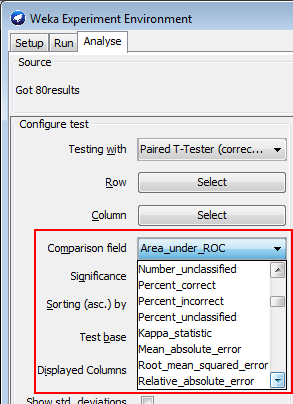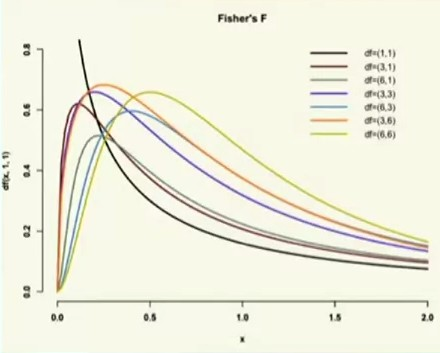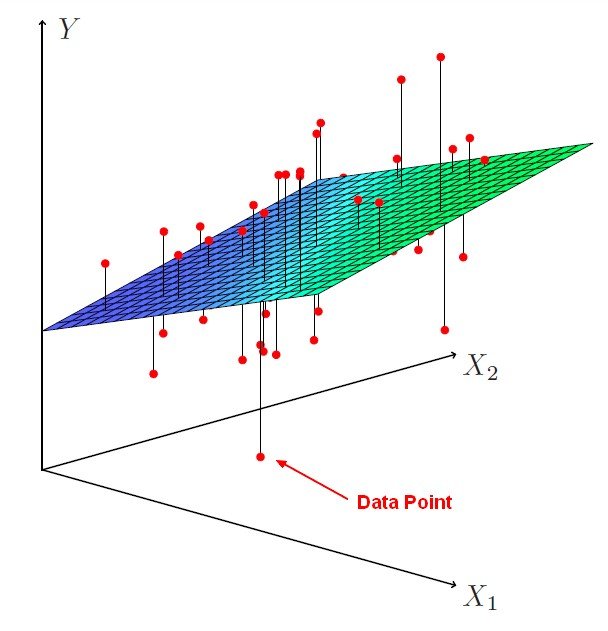# Statistics - (F-Statistic|F-test|F-ratio)

The NHST anova statistic test is an F-test or F-ratio.

It's what you observe in the numerator relative to what you would expect just due to chance in the denominator.

The f statistic is the statistic that we'll obtain if we dropped out the predictors in the model.

A lot of people refer to it as an F-ratio because it's the variance between the groups relative to variants within the groups.

An ANOVA will tell with the F-ratio if:

• there is an effect overall
• there is significant difference somewhere

The F-test has a family of F-distributions.

The F statistic tests the null hypothesis that none of the predictors has any effect. Rejecting that null means concluding that *some* predictor has an effect, not that *all* of them do.

## Formulas

$$\begin{array}{rrl} \text{F-ratio (F-test)} & = & \frac{\href{variance}{variance}\text{ between the groups}}{\href{variance}{variance}\text{ within the groups}} \\ & = & \frac{\text{systematic variance}}{\text{unsystematic variance}} \\ & = & \frac{\text{good variance}}{\text{bad variance}} \\ \end{array}$$

The variance between the groups, or across the groups, is a good variance because it was created with our independent variable (within the experimental treatment).

The variants within the groups try to determine why in one group the score of individual differ. And we don't know because that variance is unsystematic, it's just what I would expect due to chance.

If you get a ratio of two or three, you're probably going to have a significant effect with the p-value less than 0.05.

$$\begin{array}{rrl} \text{F-ratio (F-test)} & = & \frac{\href{variance}{variance}\text{ between the groups}}{\href{variance}{variance}\text{ within the groups}} \\ & = & \frac{{\href{variance}{\text{Mean Square (MS)}}}_{Betweeen}}{{\href{variance}{\text{Mean Square (MS)}}}_{Within}} \\ & = & \frac{{\href{variance}{\text{MS}}}_{A}}{{\href{variance}{\text{MS}}}_{S/A}} \\ \end{array}$$

where:

• A is the independent variable (the manipulation)
• S within A is the way to read that error term. So, it's subjects within groups

Mean square (MS) is variance

$$\begin{array}{rrl} \text{F-ratio (F-test)} & = & \frac{{\href{variance}{\text{MS}}}_{A}}{{\href{variance}{\text{MS}}}_{S/A}} \\ & = & \frac{\text{Sum of the Squares (SS)}_A}{\href{degree_of_freedom}{df}_A}. \frac{\href{degree_of_freedom}{df}_{S/A}}{\text{Sum of the Squares (SS)}_{S/A}} \\ & = & \frac{SS_A}{\href{degree_of_freedom}{df}_A}. \frac{\href{degree_of_freedom}{df}_{S/A}}{SS_{S/A}} \\ \end{array}$$ where:

• $SS_A$ will compare each group mean to the grand mean to get the variance across groups.
• $SS_{S/A}$ will look at each individual within a group and see how much they differ from their group mean.
• The $\href{degree_of_freedom}{df}_A$ is the number of group minus one.
• The $\href{degree_of_freedom}{df}_{S/A}$ is the number of subjects in a group minus one times the number of groups.

$$\begin{array}{rrl} SS_A & = & n \sum_{j=1}^{N}(Y_j - Y_T)^2 \end{array}$$

where:

• $N$ is the number of group
• $n$ is the number of subjects in each group (because if they're very large groups then we have

to take that into account)

• $Y_j$ is a group mean
• $Y_T$ is the grand mean

$$\begin{array}{rrl} SS_{S/A} & = & \sum_{j=1}^{N}\sum_{i=1}^{n}(Y_{ij} - Y_j)^2 \end{array}$$

where:

• $N$ is the number of group
• $n$ is the number of subjects in each group
• $Y_{ij}$ is an individual scores
• $Y_j$ is a group mean

Recommended PagesData Mining - (Parameters | Model) (Accuracy | Precision | Fit | Performance) Metrics

Accuracy is a evaluation metrics on how a model perform. rare event detection Hypothesis testing: t-statistic and p-value. The p value and t statistic measure how strong is the...R - Interaction Analysis

interaction with R . An interaction term between a numeric x and z is just the product of x and z. lm processes the “” operator between variables andautomatically: add the interaction...R - Multiple Linear Regression

Multiple linear regression with R functions such as lm Unstandardized Multiple Regression Regression analyses, standardized (in the z scale). The point is a short-cut to select all variables....Statistics - Analysis of variance (Anova)

Anova is just a special case of multiple regression. There're many forms of ANOVA. It's a very common procedure in basic statistics. Anova is more Appropriate when: there's true independent variable...Statistics - F-distributions

Like the t-test and family of t-distributions, the F-test has a family of F-distributions The family of F-distributions depends on: the Number of subjects per group the Number of groups ANOVA...Statistics - Factorial Anova

A factorial ANOVA is done when the independent variables are categorical. By adding a second independent variable, we are entering in factorial ANOVA. N Independent Variables (IVs). Variables that...Statistics - Multiple Linear Regression

Multiple regression is a regression with multiple predictors. It extends the simple model. You can have many predictor as you want. The power of multiple regression (with multiple predictor) is to better...Statistics - Null Hypothesis Significance Testing (NHST)

NHST is a procedure for testing the Null Hypothesis. It's a binary decision: Reject or Retain the Null Hypothesis Ie Retain the Null Hypothesis or the alternative hypothesis. Before starting any...